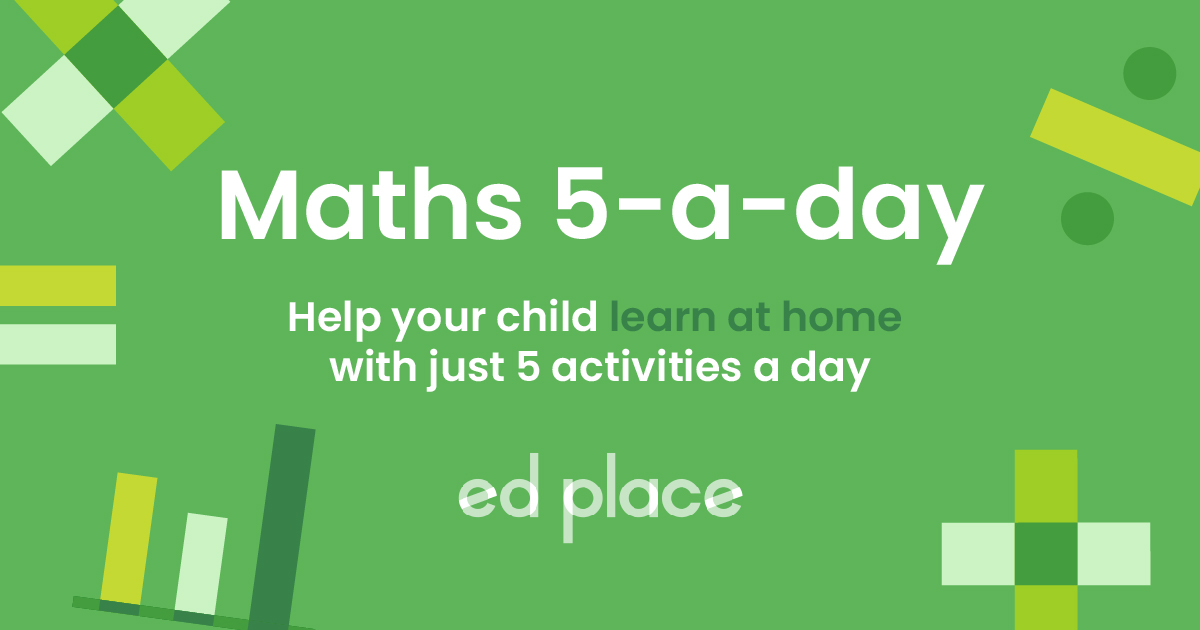# EdPlace's Year 1 and 2 home learning maths lesson: Finding Missing Numbers on a Number Line

Looking for short lessons to keep your child engaged and learning? Our experienced team of teachers have created English, maths and science lessons for the home, so your child can learn no matter where they are.  And, as all activities are self-marked, you really can encourage your child to be an independent learner.

Get them started on the lesson below and then jump into our teacher-created activities to practice what they've learnt. We've recommended five to ensure they feel secure in their knowledge - 5-a-day helps keeps the learning loss at bay (or so we think!).

Are they keen to start practising straight away? Head to the bottom of the page to find the activities.

Now...onto the lesson!

Key Stage 1 Statutory Requirements for Maths
Year 1 and 2 students should be able to identify and represent numbers using objects and pictorial representations including the number line, and use the language of: equal to, more than, less than (fewer), most, least.

Number lines are a useful way of representing numbers in Maths. They can help your child to count forwards and backwards, find an answer when calculating an addition or subtraction sum and can even be used to practice times tables. Sometimes, your child can be challenged to find the missing number on a number line, understanding how to tackle these problems will fill your child with confidence when approaching number work.

Following this step-by-step process your child will be able to:

1) Understand how to ask the right questions to find the missing number on a number line.

2) Apply this understanding to describe the steps needed to crack the problem.

3) Explain it back to you at the end!

## Step 1: The Key Terms

Before we get going, it is crucial to check your child understands the important language we will use for this objective.

Subtraction: The ‘taking away’ of one number from another to make the original number smaller. Think of ‘subtracting’ as taking apart.

Number line: Number lines are a horizontal line that visually represent numbers. They are an especially useful way to demonstrate counting, place value and basic calculation skills.

Interval: The interval on a number line is the space between two numbers and includes any numbers that fall between those two numbers.

## Step 2: Further Explanation into Key Concepts

To tackle this lesson, your child will need a sound understanding of these key concepts.

Addition: Adding two or more given numbers together to find a total, we can call this the total sum of the numbers. Initially, your child will have been taught to add using concrete objects and will then have progressed onto using a number line.

Subtraction: Subtracting allows us to find the difference between two values. Remember to subtract the smallest number from the largest. Again, your child will have been taught to subtract using concrete objects and will then have progressed onto using a number line to count backwards.

Number line: The numbers on the left of a number line are smaller and as we move along the number line to the right, the numbers increase. If we want to add, we move to the right, if we wish to subtract, we move along to the left.

## Step 3: Number Lines

When we are faced with a number line with missing numbers, we must ask ourselves 3 logical questions.

1. Which numbers have we been given?

2. Can we estimate (guess) the halfway point?

3. Can we calculate the difference between two intervals on the number line?

Look at this number line and answer the above questions.

1) We have been given numbers 0, 1, 3, 4, 6.

2) The halfway point is 3 because 3 is half of 6 and the numbers range from 0-6.

3) We can see the intervals are increasing by 1 as 3 and 4 are next to one another.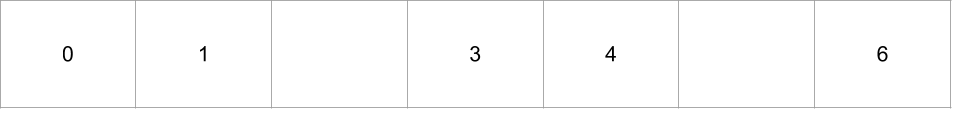What are the missing numbers? 2 and 5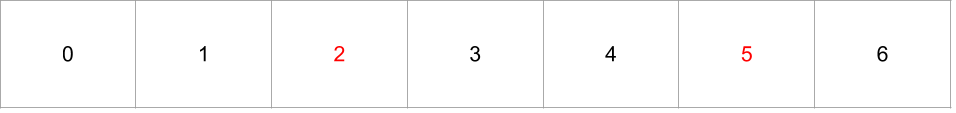Let's look at another example: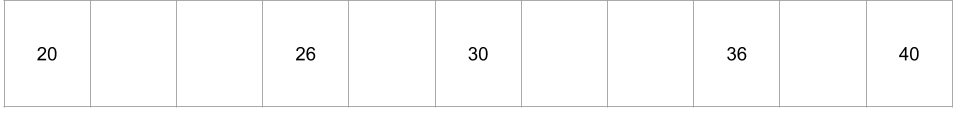Remember the 3 important questions:

1. Which numbers have we been given?

2. Can we estimate the halfway point?

3. Can we calculate the difference between two intervals on the number line?

Look at this number line and answer the above questions.

1)We have been given numbers 20, 26, 30, 36 and 40.

2)The halfway point is 30 because there is a difference of 20 between 20 and 40, 40 – 20 = 10. 20 + 10 = 30

3)We can see the intervals are increasing by 2, we can use our knowledge of the 2x table to check this.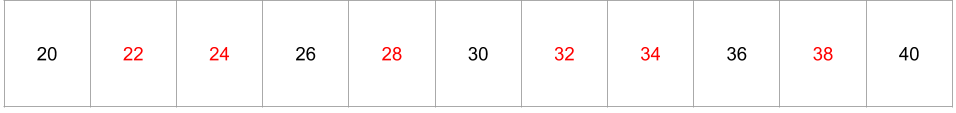## Step 4 - Putting it into Practice

Now, have a go finding the missing numbers on the number line.

a)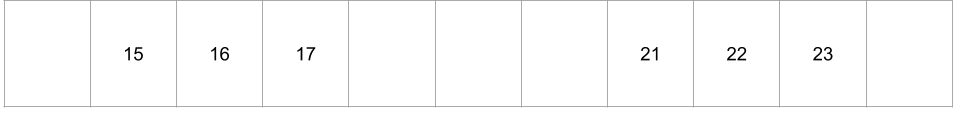b)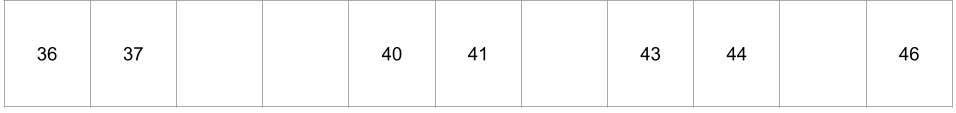c)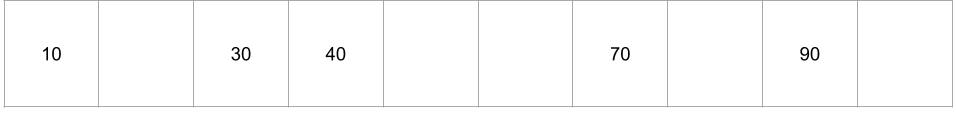d)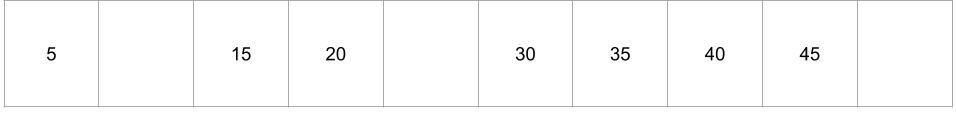## Step 5 - Give it a go...

Why not test your child's understanding and see if they can tackle these activities?

All activities are created by teachers and automatically marked. Plus, with an EdPlace subscription, we can automatically progress your child at a level that's right for them. Sending you progress reports along the way so you can track and measure progress, together - brilliant!

Step 4:

1. The missing numbers in order are: 14, 18, 19, 20, 24.

2. The missing numbers in order are: 38, 39, 42, 45.

3. The missing numbers in order are: 20, 50, 60, 80.

4. The missing numbers in order are: 10, 25, 50.

Keep going! Looking for more activities, different subjects or year groups?

Click the button below to view the EdPlace English, maths, science and 11+ activity library